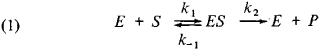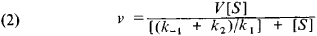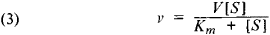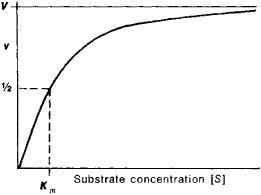# Michaelis Constant

(redirected from Michaelis, Leonor)
Also found in: Medical.

## Michaelis constant

[mi′kā·ləs ‚kän·stənt]
(biochemistry)
A constant Km such that the initial rate of reaction V, produced by an enzyme when the substrate concentration is high enough to saturate the enzyme, is related to the rate of reaction v at a lower substrate concentration c by the formula V = v (1 + Km / c).
McGraw-Hill Dictionary of Scientific & Technical Terms, 6E, Copyright © 2003 by The McGraw-Hill Companies, Inc.
The following article is from The Great Soviet Encyclopedia (1979). It might be outdated or ideologically biased.

## Michaelis Constant

one of the most important parameters of enzyme kinetics, characterizing the dependence of the rate of an enzyme process on the substrate concentration; derived by the German scientists L. Michaelis and M. Menten in 1913.

According to the Michaelis-Menten theory, the first step in any enzyme process is a reversible reaction between the enzyme (E) and the substrate (S), leading to the formation of an intermediate enzyme-substrate complex (£5), which then undergoes practically irreversible decomposition, yielding a reaction product (P) and the original enzyme (E):The reactions for the formation and decomposition of the ES complex are characterized by the rate constants k\, £_i , and k 2. If the substrate concentration markedly exceeds the enzyme concentration ( » [E]), so that the ES concentration becomes constant, then the rate of the enzyme reaction (v) is given by the equationwhere V is the maximum rate of the reaction, achieved at full saturation of the enzyme by the substrate. The ratio of the rate constants (k-\+ki)/k\ is the Michaelis constant (km).

When the Michaelis constant is substituted into equation (2), the Michaelis-Menten equation is obtained:It follows from equation (3) that the Michaelis constant is numerically equal to the substrate concentration at which the reaction rate reaches half its maximum possible value (see FigureFigure 1. Ratio of enzyme reaction rate (v) to substrate concentration [S]

In a number of cases, in which ki is negligible, the Michaelis constant becomes equivalent to k\/k \ and may serve as a measure of the affinity of the substrate for the enzyme.

The Michaelis constant is given in units of concentration. In practice, the value for the Michaelis constant is found graphically using the ratio of the enzyme reaction rate to the substrate concentration.

### REFERENCES

lakovlev, V. A. Kinetika fermentativnogo kataliza. Moscow, 1965.
Webb, L. Ingibitory fermentov i metabolizma. Moscow, 1966. (Translated from English.)

D. M. BELEN’KII